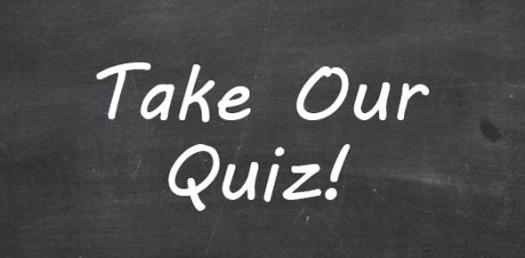8 Questions | Total Attempts: 1082Settings.

• 1.
Look at the shapes in the boxes across the rows and up and down the columns. Do you see how they are related to each other? Can you find the answer that goes in the empty box so the designs inside the rows and columns follow a pattern or rule?
• A.

A

• B.

B

• C.

C

• D.

D

• E.

E

• 2.
Which one of these would you find in a bathroom?
• A.

First Image

• B.

Second Image

• C.

Third Image

• D.

Fourth Image

• 3.
Take a look at the pictures across the top. They show a piece of paper being folded. Then, one or more holes are punched in the folded piece of paper. Can you point to the answer that shows what the square piece of paper will look like when it is unfolded?
• A.

Option 1

• B.

Option 2

• C.

Option 3

• D.

Option 4

• 4.
Look at the shapes on top. They are alike in some way and so they belong together. Choose one shape from the bottom row that belongs with the figures on top.
• A.

Boat 1

• B.

Boat 2

• C.

Boat 3

• 5.
The first two underlined words in this sentence go together in a certain way:Warts is to straw as snap is to __________.Choose the answer that goes with the third underlined word the same way the first and second underlined words go together.
• A.

Taps

• B.

Naps

• C.

Claps

• D.

Pans

• E.

Arts

• 6.
For this mathematical equation, choose the answer that should replace the X symbol:(4 + 7) + 10 = (10 + X) + 4
• A.

0

• B.

2

• C.

3

• D.

5

• E.

7

• 7.
Look at the first three figures. They go together in some way. Choose one more figure in the rest of the row that goes with the first three figures in the same way.
• A.

A

• B.

B

• C.

C

• D.

D

• E.

E

• 8.
Look at the mathematical equation to the right. Choose the answer that should replace the ?
• A.

100

• B.

1

• C.

10

• D.

80

• E.

12

Related TopicsBack to top